StatLect

Partitions into groups

A partition ofobjects intogroups is one of the possible ways of subdividing theobjects intogroups (). The rules are:

1. the order in which objects are assigned to a group does not matter;

2. each object can be assigned to only one group.

The following subsections give a slightly more formal definition of partition into groups and deal with the problem of counting the number of possible partitions into groups.Definition of partition into groups

Let,,...,beobjects. Let,, ...,be(with) groups to which we can assign theobjects.objects can be assigned to group,objects can be assigned to groupand so on.,, ...,are such that:A partition of,,...,into thegroups,, ...,is one of the possible ways to assign theobjects to thegroups.

Example Consider three objects,andand two groupsand, withThere are three possible partitions of the three objects into the two groups:Note that the order of objects belonging to a group does not matter, so, for example,in Partition 1 is the same as.

Counting the number of partitions into groups

Denote bythe number of possible partitions into thegroups (where groupcontainsobjects). How much isin general?

The numbercan be derived with the following sequential procedure:

1. First, we assignobjects to the first group. There is a total ofobjects to choose from. The number of possible ways to chooseof theobjects is equal to the number of combinations ofelements from. So there are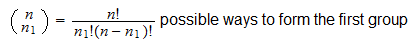2. Then, we assignobjects to the second group. There wereobjects, buthave already been assigned to the first group. So, there areobjects left, that can be assigned to the second group. The number of possible ways to chooseof the remainingobjects is equal to the number of combinations ofelements from. So there are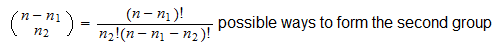and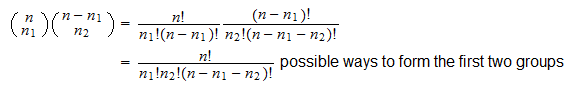3. Then, we assignobjects to the third group. There wereobjects, buthave already been assigned to the first two groups. So, there areobjects left, that can be assigned to the third group. The number of possible ways to chooseof the remainingobjects is equal to the number of combinations ofelements from. So there are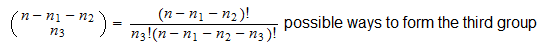and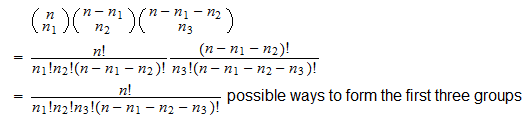4. An so on, until we are left withobjects and the last group. There is only one way to form the last group, which can also be written as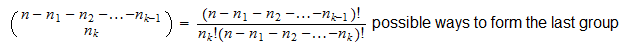Therefore, there areTherefore, by the above sequential argument, the total number of possible partitions into thegroups isThe numberis often indicated as follows:andis called a multinomial coefficient.

Sometimes the following notation is also used:Example The number of possible partitions ofobjects intogroups ofobjects is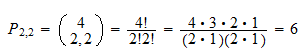More details

The following sections contain more details about partitions.

Multinomial coefficients and multinomial expansions

The multinomial coefficient is so called because it appears in the multinomial expansion:whereand the summation is over all the-tuplessuch that:Solved exercises

Below you can find some exercises with explained solutions.

Exercise 1

John has a basket of fruit containing one apple, one banana, one orange and one kiwi. He wants to give one fruit to each of his two little sisters and two fruits to his big brother. In how many different ways can he do this?

Solution

John needs to decide how to partition 4 objects into 3 groups, where the first two groups will contain one object and the third one will contain two objects. The total number of partitions isExercise 2

Ten friends want to play basketball. They need to divide into two teams of five players. In how many different ways can they do this?

Solution

They need to decide how to partition 10 objects into 2 groups, where each group will contain 5 objects. The total number of partitions isThe book

Most of the learning materials found on this website are now available in a traditional textbook format.

Glossary entries
Share